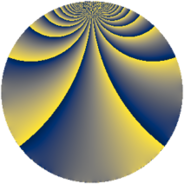Properties

 Label 567.2.fLevel $567$ Weight $2$ Character orbit 567.f Rep. character $\chi_{567}(190,\cdot)$ Character field $\Q(\zeta_{3})$ Dimension $48$ Newform subspaces $14$ Sturm bound $144$ Trace bound $10$

Related objects

Defining parameters

 Level: $$N$$ $$=$$ $$567 = 3^{4} \cdot 7$$ Weight: $$k$$ $$=$$ $$2$$ Character orbit: $$[\chi]$$ $$=$$ 567.f (of order $$3$$ and degree $$2$$) Character conductor: $$\operatorname{cond}(\chi)$$ $$=$$ $$9$$ Character field: $$\Q(\zeta_{3})$$ Newform subspaces: $$14$$ Sturm bound: $$144$$ Trace bound: $$10$$ Distinguishing $$T_p$$: $$2$$, $$5$$

Dimensions

The following table gives the dimensions of various subspaces of $$M_{2}(567, [\chi])$$.

Total New Old
Modular forms 168 48 120
Cusp forms 120 48 72
Eisenstein series 48 0 48

Trace form

 $$48 q - 24 q^{4} + O(q^{10})$$ $$48 q - 24 q^{4} - 24 q^{16} + 24 q^{19} - 12 q^{22} - 36 q^{25} - 12 q^{31} + 48 q^{34} + 24 q^{37} + 60 q^{40} - 12 q^{43} - 120 q^{46} - 24 q^{49} - 36 q^{52} + 24 q^{55} - 36 q^{58} - 24 q^{67} + 72 q^{73} + 24 q^{76} - 12 q^{79} + 72 q^{82} + 84 q^{88} + 24 q^{91} + 84 q^{94} - 36 q^{97} + O(q^{100})$$

Decomposition of $$S_{2}^{\mathrm{new}}(567, [\chi])$$ into newform subspaces

Label Dim. $$A$$ Field CM Traces $q$-expansion
$a_{2}$ $a_{3}$ $a_{5}$ $a_{7}$
567.2.f.a $2$ $4.528$ $$\Q(\sqrt{-3})$$ None $$-2$$ $$0$$ $$-1$$ $$1$$ $$q+(-2+2\zeta_{6})q^{2}-2\zeta_{6}q^{4}-\zeta_{6}q^{5}+\cdots$$
567.2.f.b $2$ $4.528$ $$\Q(\sqrt{-3})$$ None $$-1$$ $$0$$ $$-2$$ $$1$$ $$q+(-1+\zeta_{6})q^{2}+\zeta_{6}q^{4}-2\zeta_{6}q^{5}+\cdots$$
567.2.f.c $2$ $4.528$ $$\Q(\sqrt{-3})$$ None $$-1$$ $$0$$ $$1$$ $$1$$ $$q+(-1+\zeta_{6})q^{2}+\zeta_{6}q^{4}+\zeta_{6}q^{5}+(1+\cdots)q^{7}+\cdots$$
567.2.f.d $2$ $4.528$ $$\Q(\sqrt{-3})$$ None $$0$$ $$0$$ $$-3$$ $$-1$$ $$q+2\zeta_{6}q^{4}-3\zeta_{6}q^{5}+(-1+\zeta_{6})q^{7}+\cdots$$
567.2.f.e $2$ $4.528$ $$\Q(\sqrt{-3})$$ None $$0$$ $$0$$ $$3$$ $$-1$$ $$q+2\zeta_{6}q^{4}+3\zeta_{6}q^{5}+(-1+\zeta_{6})q^{7}+\cdots$$
567.2.f.f $2$ $4.528$ $$\Q(\sqrt{-3})$$ None $$1$$ $$0$$ $$-1$$ $$1$$ $$q+(1-\zeta_{6})q^{2}+\zeta_{6}q^{4}-\zeta_{6}q^{5}+(1-\zeta_{6})q^{7}+\cdots$$
567.2.f.g $2$ $4.528$ $$\Q(\sqrt{-3})$$ None $$1$$ $$0$$ $$2$$ $$1$$ $$q+(1-\zeta_{6})q^{2}+\zeta_{6}q^{4}+2\zeta_{6}q^{5}+(1+\cdots)q^{7}+\cdots$$
567.2.f.h $2$ $4.528$ $$\Q(\sqrt{-3})$$ None $$2$$ $$0$$ $$1$$ $$1$$ $$q+(2-2\zeta_{6})q^{2}-2\zeta_{6}q^{4}+\zeta_{6}q^{5}+(1+\cdots)q^{7}+\cdots$$
567.2.f.i $4$ $4.528$ $$\Q(\sqrt{-3}, \sqrt{7})$$ None $$0$$ $$0$$ $$0$$ $$2$$ $$q+\beta _{1}q^{2}+5\beta _{2}q^{4}+(\beta _{1}+\beta _{3})q^{5}+(1+\cdots)q^{7}+\cdots$$
567.2.f.j $4$ $4.528$ $$\Q(\zeta_{12})$$ None $$0$$ $$0$$ $$0$$ $$-2$$ $$q-\zeta_{12}^{2}q^{2}+(-1+\zeta_{12})q^{4}+(-2\zeta_{12}^{2}+\cdots)q^{5}+\cdots$$
567.2.f.k $4$ $4.528$ $$\Q(\zeta_{12})$$ None $$0$$ $$0$$ $$0$$ $$-2$$ $$q-\zeta_{12}^{2}q^{2}+(-1+\zeta_{12})q^{4}+(\zeta_{12}^{2}+\cdots)q^{5}+\cdots$$
567.2.f.l $6$ $4.528$ 6.0.1156923.1 None $$0$$ $$0$$ $$-3$$ $$-3$$ $$q+\beta _{4}q^{2}+(-\beta _{1}+\beta _{2}-2\beta _{3}+\beta _{4}+\cdots)q^{4}+\cdots$$
567.2.f.m $6$ $4.528$ 6.0.1156923.1 None $$0$$ $$0$$ $$3$$ $$-3$$ $$q-\beta _{4}q^{2}+(-\beta _{1}+\beta _{2}-2\beta _{3}+\beta _{4}+\cdots)q^{4}+\cdots$$
567.2.f.n $8$ $4.528$ 8.0.49787136.1 None $$0$$ $$0$$ $$0$$ $$4$$ $$q+\beta _{3}q^{2}+\beta _{4}q^{4}-2\beta _{7}q^{5}+(1-\beta _{5}+\cdots)q^{7}+\cdots$$

Decomposition of $$S_{2}^{\mathrm{old}}(567, [\chi])$$ into lower level spaces

$$S_{2}^{\mathrm{old}}(567, [\chi]) \cong$$ $$S_{2}^{\mathrm{new}}(63, [\chi])$$$$^{\oplus 3}$$$$\oplus$$$$S_{2}^{\mathrm{new}}(81, [\chi])$$$$^{\oplus 2}$$$$\oplus$$$$S_{2}^{\mathrm{new}}(189, [\chi])$$$$^{\oplus 2}$$